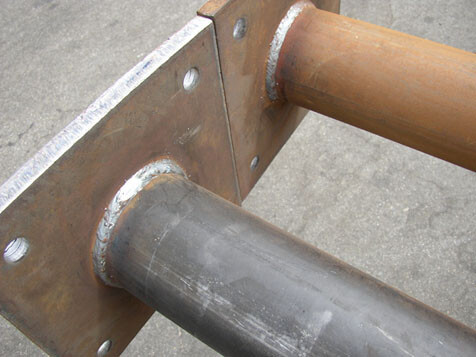# Simplified Design of Welded Connection to Eurocode 3In our previous post, we were able to present an example on the design of fillet welds for truss members using two different approaches. In this post, we are going to simplify it further by presenting an example on how the strength of fillet welds can be easily verified, according to the requirements of Eurocode 3.

How to Design Fillet Weld Connection for Steel Trusses

Solved Example
A 150 mm diameter hollow steel pipe is welded to a plate of thickness 25 mm of grade S275. If the welding side is 12 mm, determine the shear resistance of the welded connection (see connection example with image above).

Solution
Thickness of plate (t) = 25 mm < 40 mm, therefore fu = 430 Mpa (Table 3.1 BS EN 1993-1-1)

For steel grade S275, βw = 0.85 (Table 4.1, BS EN 1993-1-1)

The throat of the weld
a = (√2/2) × welding side
a = 0.7071t
a = 0.7071(12) = 8.485 mm

Design weld shear strength
fvwd = (fu/√3)/(βwγm2)
fvwd = (430/√3)/(0.85 × 1.25) = 233.65 Mpa

Weld resistance per length
Fw,Ed =   fvwda
Fw,Ed =  233.65 × 8.485 = 1982.58 N/mm = 1.982 kN/mm

Since the weld is done right round the perimeter of the pipe, the weld length L = πd = π × 150 = 471.24 mm

The total weld resistance = Fw,Ed × L = 1.982 × 471.24 = 934 kN

Therefore, the shear force at the base must not exceed 934 kN